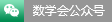Some recent domestic mathematical activities (Sep19 - Sep25, 2021)

Date:2021.09.19-2021.09.25

Introduction:Some recent domestic mathematical activities (Sep19 - Sep25, 2021). The information is taken from Tianyuan Mathematical Center in Northeast China.

Some recent domestic mathematical activities (Sep12 - Sep18, 2021)

Date:2021.09.12-2021.09.18

Introduction:Some recent domestic mathematical activities (Sep12 - Sep18, 2021). The information is taken from Tianyuan Mathematical Center in Northeast China.

Some recent domestic mathematical activities (Sep5 - Sep11, 2021)

Date:2021.09.05-2021.09.11

Introduction:Some recent domestic mathematical activities (Sep5 - Sep11, 2021). The information is taken from Tianyuan Mathematical Center in Northeast China.

Some recent domestic mathematical activities (Aug29 - Sep4, 2021)

Date:2021.08.29-2021.09.04

Introduction:Some recent domestic mathematical activities (Aug29 - Sep4, 2021). The information is taken from Tianyuan Mathematical Center in Northeast China.

Some recent domestic mathematical activities (Aug23 - Aug29, 2021)

Date:2021.08.23-2021.08.29

Introduction:Some recent domestic mathematical activities (Aug23 - Aug29, 2021). The information is taken from Tianyuan Mathematical Center in Northeast China.

Some recent domestic mathematical activities (Aug16 - Aug23, 2021)

Date:2021.08.16-2021.08.23

Introduction:Some recent domestic mathematical activities (Aug16 - Aug23, 2021). The information is taken from Tianyuan Mathematical Center in Northeast China.

Some recent domestic mathematical activities (Aug9 - Aug16, 2021)

Date:2021.08.09-2021.08.16

Introduction:Some recent domestic mathematical activities (Aug9 - Aug16, 2021). The information is taken from Tianyuan Mathematical Center in Northeast China.

Some recent domestic mathematical activities (Aug2 - Aug8, 2021)

Date:2021.08.02-2021.08.08

Introduction:Some recent domestic mathematical activities (Aug2 - Aug8, 2021). The information is taken from Tianyuan Mathematical Center in Northeast China.

Some recent domestic mathematical activities (Jul26 - Aug1, 2021)

Date:2021.07.26-2021.08.01

Introduction:Some recent domestic mathematical activities (Jul26 - Aug1, 2021). The information is taken from Tianyuan Mathematical Center in Northeast China.

Some recent domestic mathematical activities (Jul19 - Jul25, 2021)

Date:2021.07.19-2021.07.25

Introduction:Some recent domestic mathematical activities (Jul19 - Jul25, 2021). The information is taken from Tianyuan Mathematical Center in Northeast China.

Some recent domestic mathematical activities (Jul12 - Jul18, 2021)

Date:2021.07.12-2021.07.18

Introduction:Some recent domestic mathematical activities (Jul12 - Jul18, 2021). The information is taken from Tianyuan Mathematical Center in Northeast China.

Some recent domestic mathematical activities (Jul4 - Jul11, 2021)

Date:2021.07.04-2021.07.11

Introduction:Some recent domestic mathematical activities (Jul4 - Jul11, 2021). The information is taken from Tianyuan Mathematical Center in Northeast China.

Some recent domestic mathematical activities (Jun28 - Jul4, 2021)

Date:2021.06.28-2021.07.04

Introduction:Some recent domestic mathematical activities (Jun28 - Jul4, 2021). The information is taken from Tianyuan Mathematical Center in Northeast China.

Mathematics without Borders: The Centennial of the International Mathematical Union

Date:2021.09.27-2021.09.28

Introduction:Mathematics without Borders: The Centennial of the International Mathematical Union September 27-28 2021, Palais Universitaire, Strasbourg

Some recent domestic mathematical activities (Jun21 - Jun27, 2021)

Date:2021.06.21-2021.06.27

Introduction:Some recent domestic mathematical activities (Jun21 - Jun27, 2021). The information is taken from Tianyuan Mathematical Center in Northeast China.

#### Professor Hua Loo-Keng is a famous mathematician

Professor Hua Loo-Keng is a famous Chinese mathematician and loves his motherland deeply. He devoted into the scientific career, and made an outstanding contribution to the Chinese mathematical career and talents cultivation for all his life.

#### Prof Chern is a worldwide math master

As a Chinese American mathematician, a foreign academician of Chinese Academy of Sciences, Professor Shiing Shen Chern is a worldwide math master. He made outstanding contributions to the development of mathematics.

#### Professor Jiaqing ZHONG

Professor Jiaqing ZHONG was very concerned about the development of the mathematics of the motherland during his lifetime and worked hard for it. He has said that the development of mathematics depends on the active cultivation and selection of outstanding young mathematicians. He sincerely wish to establish a fund in China to reward outstanding young mathematicians.#### WeChat

Scan two-dimensional code attention